Logarithmic Differentiation - Type 2

Chapter 5 Class 12 Continuity and Differentiability
Concept wise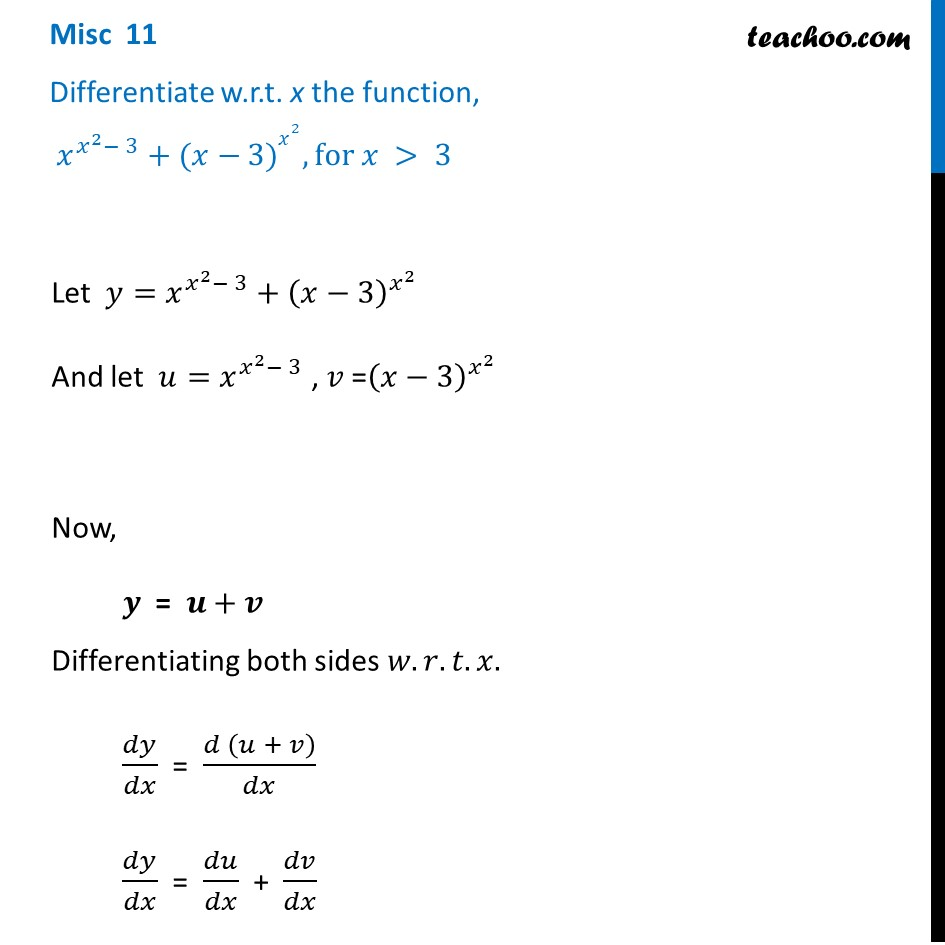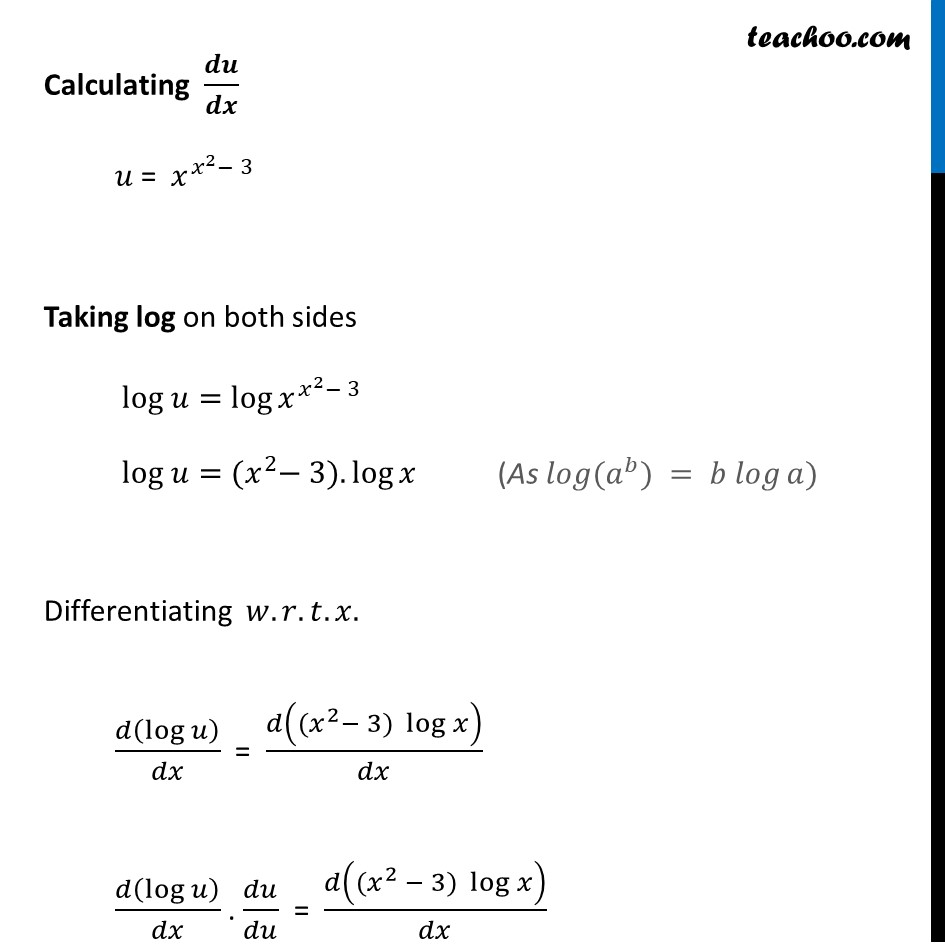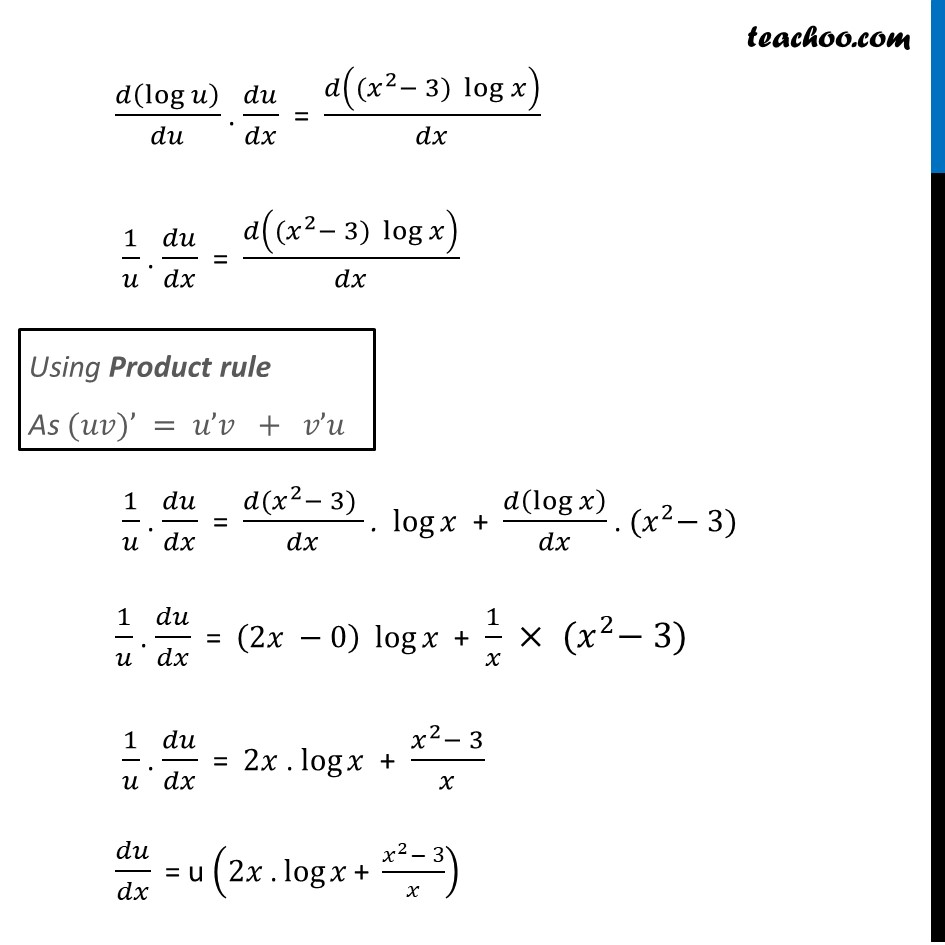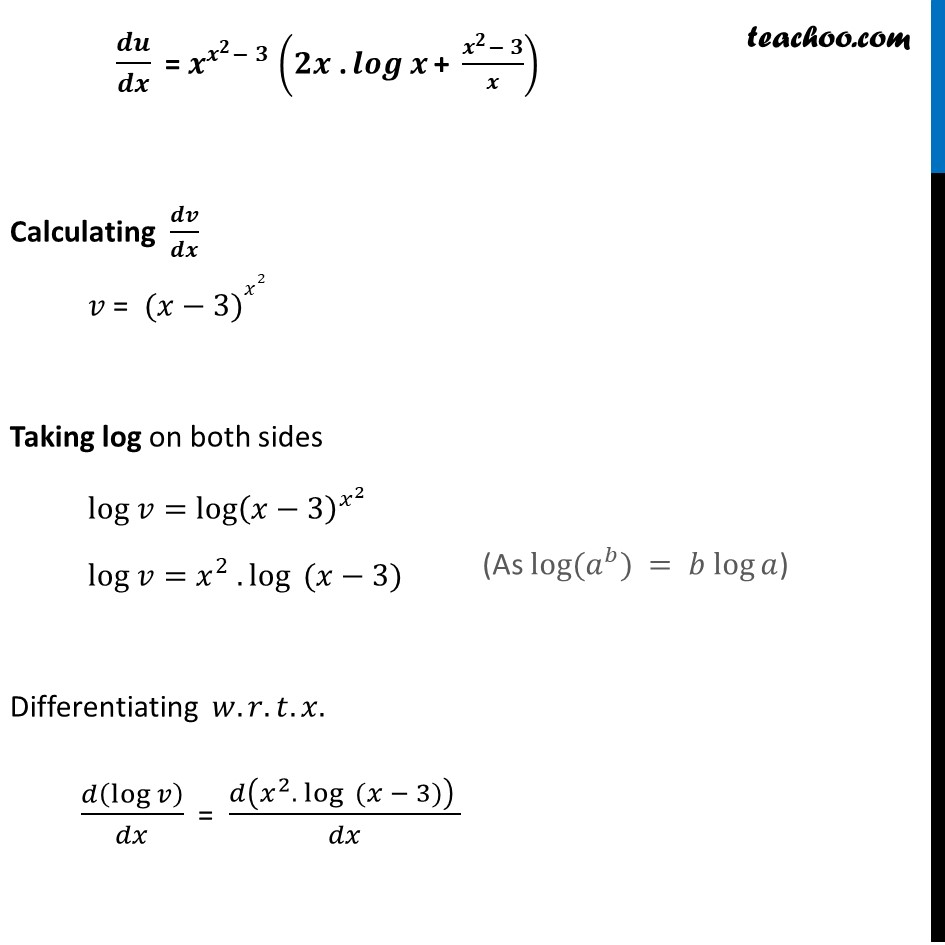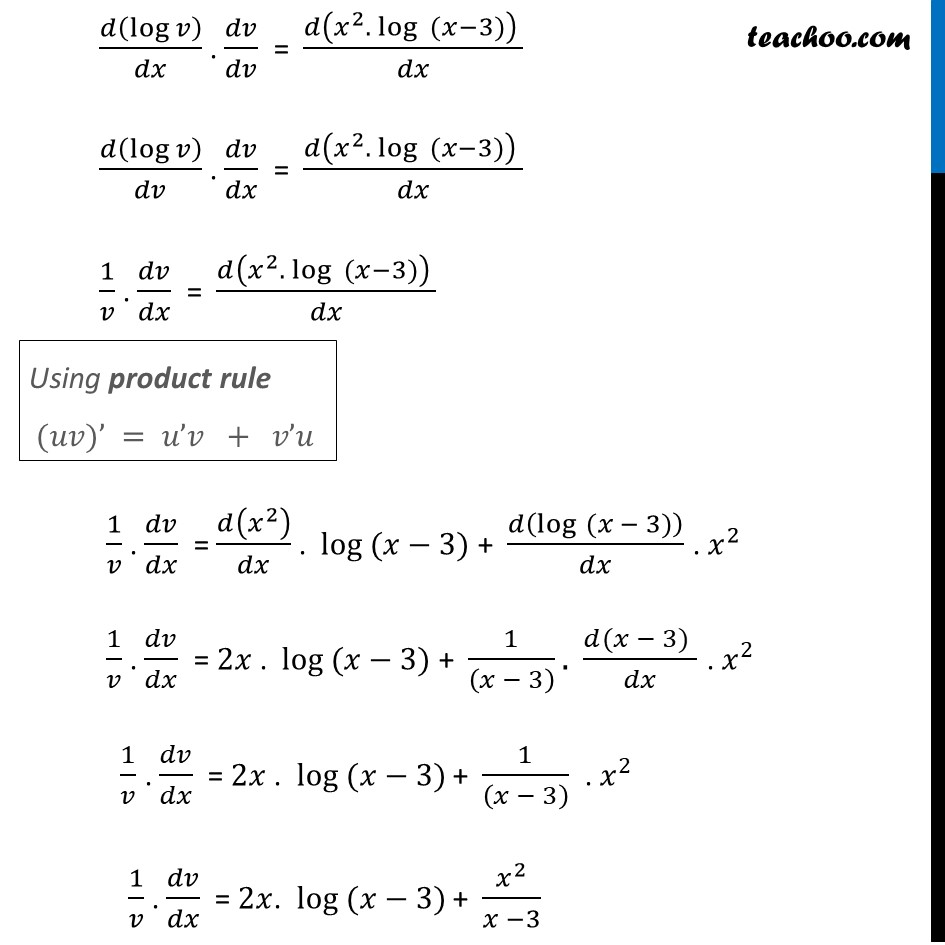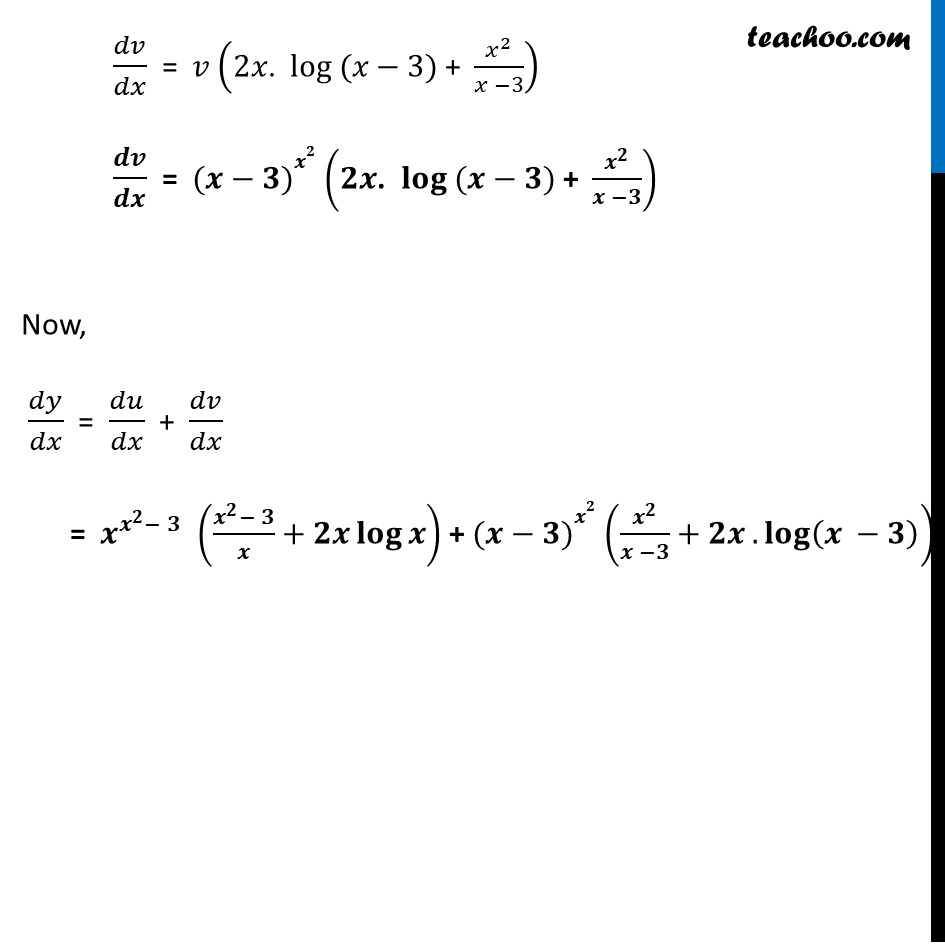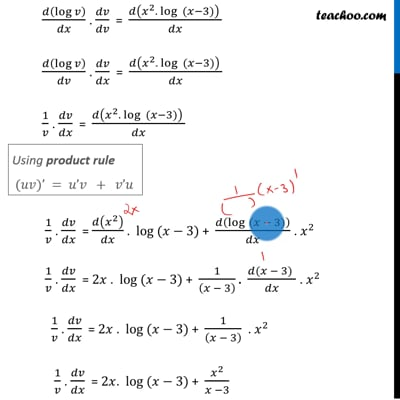This video is only available for Teachoo black users

Introducing your new favourite teacher - Teachoo Black, at only ₹83 per month

### Transcript

Misc 11 Differentiate w.r.t. x the function, 𝑥^(𝑥^2− 3)+(𝑥−3)𝑥^2, for 𝑥 > 3Let 𝑦=𝑥^(𝑥^2− 3)+(𝑥−3)^(𝑥^2 ) And let 𝑢=𝑥^(𝑥^2− 3) , 𝑣 =(𝑥−3)^(𝑥^2 ) Now, 𝒚 = 𝒖+𝒗 Differentiating both sides 𝑤.𝑟.𝑡.𝑥. 𝑑𝑦/𝑑𝑥 = (𝑑 (𝑢 + 𝑣))/𝑑𝑥 𝑑𝑦/𝑑𝑥 = 𝑑𝑢/𝑑𝑥 + 𝑑𝑣/𝑑𝑥 Calculating 𝒅𝒖/𝒅𝒙 𝑢 = 𝑥^(𝑥^2− 3) Taking log on both sides log 𝑢=log⁡〖𝑥^(𝑥^2− 3) 〗 log 𝑢=〖(𝑥〗^2− 3). log⁡𝑥 Differentiating 𝑤.𝑟.𝑡.𝑥. 𝑑(log⁡𝑢 )/𝑑𝑥 = 𝑑(〖(𝑥〗^2− 3) log⁡𝑥 )/𝑑𝑥 𝑑(log⁡𝑢 )/𝑑𝑥 . 𝑑𝑢/𝑑𝑢 = 𝑑(〖(𝑥〗^2 − 3) log⁡𝑥 )/𝑑𝑥 " " (As 𝑙𝑜𝑔⁡(𝑎^𝑏) = 𝑏 𝑙𝑜𝑔⁡𝑎) 𝑑(log⁡𝑢 )/𝑑𝑢 . 𝑑𝑢/𝑑𝑥 = 𝑑(〖(𝑥〗^2− 3) log⁡𝑥 )/𝑑𝑥 " " 1/𝑢 . 𝑑𝑢/𝑑𝑥 = 𝑑(〖(𝑥〗^2− 3) log⁡𝑥 )/𝑑𝑥 1/𝑢 . 𝑑𝑢/𝑑𝑥 = (𝑑〖(𝑥〗^2− 3) )/𝑑𝑥 . 〖 log〗⁡𝑥 + 𝑑(log⁡𝑥 )/𝑑𝑥 . 〖(𝑥〗^2− 3) 1/𝑢 . 𝑑𝑢/𝑑𝑥 = (2𝑥 −0) 〖 log〗⁡𝑥 + 1/𝑥 × 〖(𝑥〗^2− 3) 1/𝑢 . 𝑑𝑢/𝑑𝑥 = 2𝑥 . log⁡𝑥 + (𝑥^2− 3)/𝑥 𝑑𝑢/𝑑𝑥 = u (2𝑥 "." log⁡𝑥 "+ " (𝑥^2− 3)/𝑥) Using Product rule As (𝑢𝑣)’ = 𝑢’𝑣 + 𝑣’𝑢 𝒅𝒖/𝒅𝒙 = 𝒙^(𝒙^𝟐− 𝟑) (𝟐𝒙 "." 𝒍𝒐𝒈⁡𝒙 "+ " (𝒙^𝟐− 𝟑)/𝒙) Calculating 𝒅𝒗/𝒅𝒙 𝑣 = (𝑥−3)𝑥^2 Taking log on both sides log 𝑣=log⁡〖(𝑥−3)^(𝑥^2 ) 〗 log 𝑣=〖𝑥^2 . log〗⁡〖 (𝑥−3)〗 Differentiating 𝑤.𝑟.𝑡.𝑥. 𝑑(log⁡𝑣 )/𝑑𝑥 = (𝑑(〖𝑥^2. log〗⁡〖 (𝑥 − 3)〗 ) )/𝑑𝑥 (As log⁡(𝑎^𝑏) = 𝑏 log⁡𝑎) 𝑑(log⁡𝑣 )/𝑑𝑥 . 𝑑𝑣/𝑑𝑣 = (𝑑(〖𝑥^2. log〗⁡〖 (𝑥−3)〗 ) )/𝑑𝑥 𝑑(log⁡𝑣 )/𝑑𝑣 . 𝑑𝑣/𝑑𝑥 = (𝑑(〖𝑥^2. log〗⁡〖 (𝑥−3)〗 ) )/𝑑𝑥 1/𝑣 . 𝑑𝑣/𝑑𝑥 = (𝑑(〖𝑥^2. log〗⁡〖 (𝑥−3)〗 ) )/𝑑𝑥 1/𝑣 . 𝑑𝑣/𝑑𝑥 = 𝑑(𝑥^2 )/𝑑𝑥 . log (𝑥−3) + 𝑑(log" " (𝑥 − 3))/𝑑𝑥 . 𝑥^2 1/𝑣 . 𝑑𝑣/𝑑𝑥 = 2𝑥 . log (𝑥−3) + 1/((𝑥 − 3) ). (𝑑(𝑥 − 3)" " )/𝑑𝑥 . 𝑥^2 1/𝑣 . 𝑑𝑣/𝑑𝑥 = 2𝑥 . log (𝑥−3) + 1/((𝑥 − 3) ) . 𝑥^2 1/𝑣 . 𝑑𝑣/𝑑𝑥 = 2𝑥. log (𝑥−3) + 𝑥^2/(𝑥 −3)Using product rule (𝑢𝑣)’ = 𝑢’𝑣 + 𝑣’𝑢 𝑑𝑣/𝑑𝑥 = 𝑣 (2𝑥". " log" " (𝑥−3)" + " 𝑥^2/(𝑥 −3)) 𝒅𝒗/𝒅𝒙 = (𝒙−𝟑)𝒙^𝟐 (𝟐𝒙". " 𝐥𝐨𝐠" " (𝒙−𝟑)" + " 𝒙^𝟐/(𝒙 −𝟑)) Now, 𝑑𝑦/𝑑𝑥 = 𝑑𝑢/𝑑𝑥 + 𝑑𝑣/𝑑𝑥 = 𝒙^(𝒙^𝟐− 𝟑) ((𝒙^𝟐− 𝟑)/𝒙+𝟐𝒙 𝐥𝐨𝐠⁡𝒙 ) + (𝒙−𝟑)𝒙^𝟐 (𝒙^𝟐/(𝒙 −𝟑)+𝟐𝒙 .𝐥𝐨𝐠⁡(𝒙 −𝟑) )